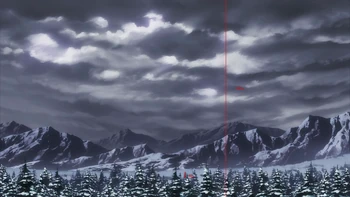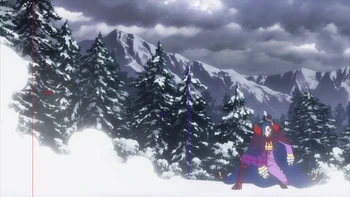## FANDOM

24,789 Pages

So this is my attempt at getting anything from this feat. Basically Ros and Puck went full-blown DBZ on one another and produced an explosion that reaches all the way to the camera so you can't exactly meassure it but I'll still try.Let's begin by finding the distance of the first trees that get nuked away from the camera. These are fir trees that range from 10 to 80 m so we'll go the average of 45 m

Frame height - 1080px

Tree height - 36px

Distance = 45*1080/[36*2*tan(70deg/2)] = 1424.61 m

Now for how far away Roswaal and subsequently the explosion are.

Ros is 1.86 m and 530.87px

Distance = 1.86*1080/[530.87*2*tan(70deg/2)] = 3.99 m

And the distance between the trees and the camera.

Distance = 45*1080/[625*2*tan(70deg/2)] = 82.05 m

Distance between Ros and the trees - 78.06 m

Ros is 78.06 m away from the trees that are 1424.61 m away from the camera.

Radius of the explosion - 1502.67 m

Now for the crater that would leave, it will have the shape of a hemisphere and since explosion's center is at most a few meters above ground we shall take the radius as the height of the hemisphere.

Plugging it into a hemisphere calculator we get--

Volume - 7106396934474578 cm^3

IF Pulverization - 7106396934474578*214 = 1.520769e+18 J

IF Vaporization - 7106396934474578*27050 = 1.92228e+20 J

Alright, after consulting with DMUA we agreed to use the nuke map calculator (read comments to see why). What we get is about 1.565 Megatons of TNT or Low 7-B give or take 1 kiloton.

Community content is available under CC-BY-SA unless otherwise noted.